# Tutorial on Introduction to biostatistics

Statistics can be defined as the science of collecting, organizing, analyzing and interpreting data - when applied to biological problems, it is known as biostatistics. The role of biostatistics is vital in every stage from research conception to the final analysis. A minimum knowledge of biostatistics is essential to be a successful researcher. This chapter examines basic biostatistical principles and explores their application with relevant examples.

Need for biostatistical tools

Biostatistical tools are necessary in research since it is almost impossible to study an entire population because of the scarcity of resources such as money, time etc or due to a desire to expose only the minimal number of subjects to the risks involved with certain clinical trials. When an entire population cannot be studied, then a part of the population (i.e. sample) is examined.

When the researcher studies the sample and draws inferences about the population, serious errors can result if the sample is not truly representative of the larger population.

Biostatistical tools help the researcher to overcome this bias and help draw valid conclusions about the population with a defined level of confidence. Biostatistics has a role in each phase of the research. Let us start with the first stage of research - planning.

Use of biostatistical tools in planning research

After identifying and defining the problem, the researcher decides on the type of study design to follow. Once the type is determined, the variables have to be identified and classified before proceeding to the next step of developing a hypothesis in the case of experimental study. The variables can be categorized by their nature, type and scales of measurement, i.e. independent or dependent, quantitative or qualitative, types such as nominal, ordinal, interval and ratio scales and so on. The nature of the variables will have a significant effect on data collection and analysis.

Variable classification according to their nature

a.      Independent variables

Variables that can either be manipulated by the researcher or which are not outcomes of the study but still affect its results are called independent variables. A good example is age of the subjects, which can be an independent variable that may affect a study outcome variable such as subject survival.

b.      Dependent variables

The outcome variables defined as part of the research process are termed dependent variables and these will be affected by the independent variables under study. An example of a dependant variable can be the number of people who developed a particular disease in a cohort study.

Variable classification according to their type

a.      Quantitative variables

Variables that can be measured numerically are called quantitative variables. These variables can be further classified as continuous and discrete variables. A continuous variable could take any value in an interval. Examples of continuous data are findings for measurements like body mass, height, blood pressure or serum cholesterol.

Discrete variables will have whole integer values. Examples are the number of hospitalizations per patient in a year or the number of hypoglycemic events recorded in a diabetic patient over 6 months.

b.      Qualitative variables

Variables, which cannot be measured numerically, are called qualitative variables. An example is gender.

Variable classification according to the scale of measurement

a.      Nominal Scale variables

Nominal scale measurements can only be classified but not put into an order, and mathematical functions cannot be performed on them. Gender is an example for this sort of variable as well.

b.      Ordinal Scale Variables

These variables can be put into a definite order, but the difference between two positions in the ordinal scale does not have a quantitative meaning. Essentially, this scale is a form of ranking. An example is the military hierarchy, where a general outranks a colonel who in turn outranks a captain. Though there is a clear series of ranks, the relationship is not numerical.
c. Interval Scale Variables

In an interval measurement scale, one unit on the scale represents the same magnitude of the characteristic being measured across the whole range of the scale, i.e. the intervals between the numbers are equal. However, the ratio between set of two numbers in the scale are not equal because an interval scale lacks a true zero point.

Temperature in Fahrenheit would be a perfect example for interval scales because though we can add and subtract degrees (70° is 10° warmer than 60°), we cannot multiply values or create ratios (70° is not twice as warm as 35°).

d.      Ratio Scale Variables

Ratio scale variables will have all the properties of interval variables with the ratio between two numbers in the ratio scales being identical. Ratio scales have an absolute or zero point. For example, a 100-year old person is indeed twice as old as a 50-year old one

### Statistical Hypothesis

After identifying and defining the variables to be investigated, the researcher has to develop the study hypothesis if conducting an experimental study. Classically, such studies will have two hypotheses. One is a null hypothesis, which is a statement of no effect or no association while the alternative hypothesis is a statement that depicts the researcher’s interest or scientific belief.

To illustrate, suppose a researcher wants to test whether a form of chemotherapy for treating small cell lung cancer is more effective than the standard therapy. The researcher can formulate the null and alternative hypothesis as follows:

Null Hypothesis:

There is no difference in efficacy between the standard therapy and the new therapy

Alternative Hypothesis:

New therapy is superior to the standard therapy.

Two types of errors can occur while making conclusions regarding the null hypothesis: Type I error and Type II error. A Type I error refers to rejecting the null hypothesis when the null hypothesis is true (false positive). A Type II error refers to accepting the null hypothesis when it is actually false (false negative).

Level of Significance and Power of the Test

The probability of making a Type I error is called level of significance (α). Normally researchers would aim to minimize the probability of making a Type I error. Most researchers will set this probability to 0.05.

The probability of making a Type II error is (β). The power of the study is calculated from (1-β) and is defined as the probability of detecting a real difference when the null hypothesis is false.

These parameters have to be predetermined by the researcher prior to the study to avert the risk of erroneously accepting the null hypothesis (even though it is really false) due to an inadequate sample size that is not enough to detect a true difference.

Once the hypothesis, level of significance and power of the study have been fixed, the researcher can proceed to determine the statistical processes for the proper conduct and analysis of the study.

sampling

As discussed earlier, the researcher usually draws conclusions about the population from a small part of it – the sample. The information collected from the sample is known as sample statistics which is used to estimate the characteristics of the unknown population i.e. population parameters.

We know that the sample taken from the population should accurately represent the population under study. To get a representative sample, the most important intervention is to select a sample large enough to adequately represent the population. Sadly, researchers have to strike a balance between striving for maximal validity while keeping the cost of the study at a level they can afford...!

From what has been stated so far, it can be deduced that the sampling process involves two important aspects. One is deciding the

Method of sampling

Method of sampling involves selection of samples from the given population. There are two basic methods in sampling.

a)       Probability sampling

b)    Non-probability  sampling

a)Probability Sampling

(i)                  Simple Random Sampling

(ii)                Stratified Random Sampling

(iii)               Systematic sampling

(iv)              Cluster sampling

b)Non Probability Sampling

(i)                  Judgement sampling

(ii)                Convenience sampling

Determining the sample size is based of a number of issues such as:

• Type of study
• Nature of study i.e. whether estimating parameters or comparing parameters
• Type of sampling method
• Type of analysis used in the study
• Power of the study
• Effect size
• Study budget
• Time factor

It can be readily appreciated that sample size calculation is rather complex. It is always best to consult a statistician for determination of sample size and other challenging biostatistical issues if embarking on a research project.

## Data Collection

After determining the sample size the researcher then proceeds to collect data. Data can be gathered through primary or secondary sources.

Primary Sources

Primary sources are original materials collected by the investigator himself. While collecting the primary data, the researcher can use the following methods.

·        Personal interview

·        Telephone interview

·        Face to face administration of questionnaire

·        Mailing questionnaire by post

·        Mailing questionnaire by email

·        Online data collection through websites

Each of the above methods has its advantages and limitations. A rule of thumb is to verify 5% of the data as a quality control measure to validate the data.

Secondary sources

Secondary data is that which has been collected by individuals or agencies for purposes other than those of our particular research study. For example, if a government department has conducted a survey of, say, family health expenditures, then a health insurance company might use this data in the organization’s evaluations of the total potential market for a new insurance product.

# Examples of secondary sources are

• Bibliographies
• Online databases
• Biographies
• Textbooks
• Handbooks and manuals
• Review articles and editorials

# Data compilation

Once the data is collected and validated it can then be compiled. Tabulation is the basic method of compilation.

# Data Analysis

Primary data analysis starts with the diagrammatic and graphical representation of the data. The following are frequently used methods:

A. Histogram

B .Bar Graph

C .Pie chart

D. Line graph

# A. Histogram

Histograms consist of a series of blocks or bars, each with an area proportional to the frequency.  In a histogram the horizontal scale is used for the variable and the vertical scale to show the frequency.

The highest block in a histogram indicates the most frequent values. The lowest blocks show the least frequent values.  Where there are no blocks, there are no results that correspond to those values.  Blocks of equal height indicate that the values they represent occur in the same frequency.

Age distribution of patients in a cancer study# B. Bar Graph

In a simple bar chart, each bar represents a different group of data. Although the bars may be drawn either vertically or horizontally, it is conventional to draw the bars vertically whenever possible. The height or length of the bar is drawn in proportion to the size of the group of data being represented.  Unlike a histogram, the bars are drawn separated from one another.

Sex wise distribution of patients in a cancer study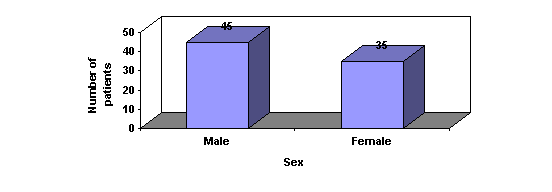# C. Pie Charts

Pie charts, or circle graphs as they are sometimes known, are very different from other types of graphs. They don't use a set of axes to plot points.  Pie charts display percentages.

The circle of a pie graph represents 100%. Each portion that takes up space within the circle stands for a part of that 100%. In this way, it is possible to see how something is divided among different groups.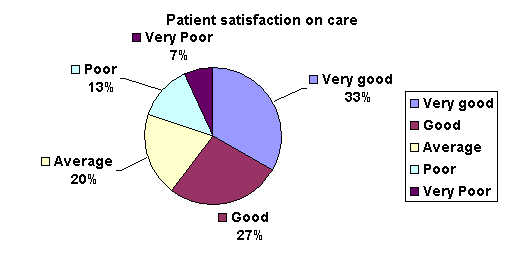# D. Line graph

A Line graph is drawn after plotting points on a graph that are then connected by a line. Line graphs are useful to display data trends.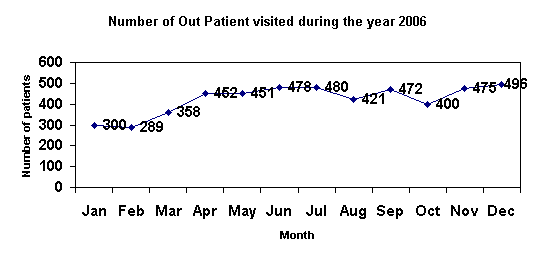Descriptive data analysis

The next stage of data analysis consists of descriptive and inferential data analysis. Descriptive data analysis provides the researcher a basic picture of the problem he is studying. It consists of:

§         Measures of Central Tendency

§         Measures of Skewness and Kurtosis

§         Measures of Dispersion.

### Measures of central tendency

A measure of central tendency is a value that represents a typical or central element of a data set. The important measures of central tendency are

a) Mean

b) Median

c) Mode

# Mean

Mean (average) is the sum of the data entries divided by the number of entries.Sample mean is denoted by    X   and the population mean is denoted by μ.

Population Mean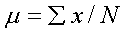Where N is the number of items in the population

# Sample Mean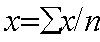Where n is the number of items in the sample

Properties of Mean

•Data possessing an interval scale or a ratio scale, usually have a mean.

•All the values are included in computing the mean.

•A given set of data has a unique mean.

•The mean is affected by unusually large or small data values (known as outliers).

•The arithmetic mean is the only measure of central tendency where the sum of the deviations of each value from the mean is zero.

# Median

The median of a data set is the middle data entry when the data set is sorted in order.   If the data set contains an even number of elements, the median is the mean of the two middle entries. The median is the most appropriate measure of central tendency to use when the data under consideration are ranked data, rather than quantitative data

Mode

The mode of a data set is the entry that occurs with the greatest frequency.  A set may have no mode or may be bimodal when two entries each occur with the same greatest frequency. The mode is most useful when an important aspect of describing the data involves determining the number of times each value occurs.

If the data are qualitative then mode is particularly useful

The following table gives you an overview of which measure of central tendency is appropriate for each data measurement scale: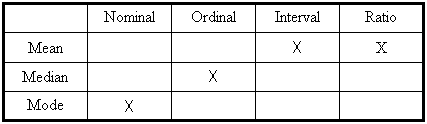Measures of Dispersion

Measures of Dispersion indicate the amount of variation or spread, in a data set.  There are four important measures of dispersion.

a)      Range

b)      Interquartile Range

c)      Variation

d)      Standard Deviation

a) The Range

The range is the difference between the largest and smallest observation.

The range is very sensitive to extreme values because it uses only the extreme values on each end of the ordered array. The range completely ignores the distribution of data.

The Interquartile Range

The interquartile range (midspread) is the difference between the

third and first quartiles.

Interquartile range         =          Q3        -           Q1

The interquartile range:
• Gives the range of the middle 50% of the data
• Is not affected by extreme values
• Ignores the distribution of data within the sample

Variance

The variance is the average of the squared differences between each observation and the mean.

Standard deviation

Standard deviation is the square root of the sample variance.

Properties of standard deviation

It lends itself to further mathematical analysis in a way that the range cannot because the standard deviation can be used in calculating other statistics.

It is worth noting that the standard deviation for nominal or ordinal data cannot be measured because it is not possible to calculate a mean for such data.

Relationships between two variables

Two of the important techniques used to study the relationship between two variables are correlation and regression.

Correlation

·        Measures association between two variables

·        In graph form it would be shown as a ‘scatter diagram’ putting the scores for one variable on the horizontal X axis and the values for the other variable on the vertical Y axis.

·        The pattern shows the strength of the association between the two variables and also whether it is a ‘positive’ or ‘negative’ relationship.

·         A ‘positive’ relationship means that as the value on one variable increases so does the value on the other variable.

·        A ‘negative’ relationship means that as the value on one variable increases, the value on the other variable decreases.

# Measures of correlation

There are two measures of correlation. One is Pearson’s product- moment correlation - r and other is Spearman’s rank order co-efficient – rho. Both the measures will tell us only how closely the two variables are connected but they cannot tell us whether one causes the other. Correlation values can range from –1 to +1

Interpretation of correlation value

Equal to 0                                 no correlation

Less than .2                              is very low

Between .2 and .4                   is low

Between .41 and .70                is moderate

Between .71 and .90                is high

Over .91                                  is very high

Equal to 1                                 perfect correlation

# Scatter diagrams can also be used to depict the correlation between two variables. The greater the spread/scatter, the less will bethe correlation value. The following scatter diagram shows the correlation between age and weight in a cancer study.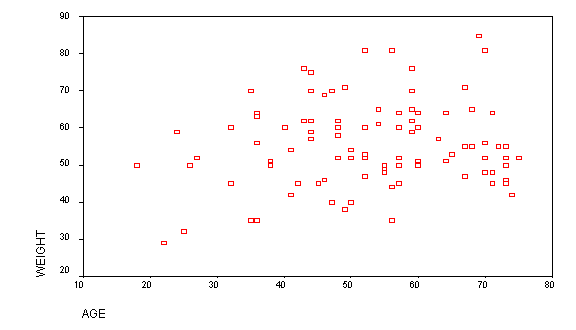From merely inspecting the diagram we can infer that there is low correlation because the spread is large while the location of the scatter plot towards the upper right tells us that whatever correlation may exist is likely to be positive. The Pearson correlation coefficient for the same data was determined to be 0.196, which confirms a very low positive correlation.

# Properties of correlation

• Correlation will not establish a cause and effect relationship
• Correlation may sometimes be a non-sense correlation
• It is very sensitive to extreme values

Simple Regression analysis:

It gives the equation of a straight line and enables prediction of one variable value from the other. Normally, the dependent variable is plotted on the Y axis and the independent variable on the X axis. There are 3 major assumptions - first, any value of x and y are normally distributed. Second, the variability of y should be the same for each value of y. Third; the relationship between the two variables is linear.

The equation of a regression line is: “y=a + bx”  where ‘a’ is the intercept, ‘b’ is the slope, ‘x’ is the independent variable and ‘y’ is the dependent variable. The slope ‘b’ is sometimes called regression coefficient and it has the same sign as correlation co-efficient (i.e., ‘r’).

Probability

Probability is defined as the likelihood of an event or outcome in trial.

p(A) =    Number of outcomes classified as A

Total number of possible outcomes

### Statistical Distributions

Statistical distributions are classified into two categories - discrete and continuous.

# Discrete distributions

Binomial distribution

It describes the possible number of times that a particular event will occur in a sequence of observations. The event is coded in binary fashion; it may or may not occur. The binomial distribution is used when a researcher is interested in the occurrence of an event, not in its magnitude. For instance, in a clinical trial, a patient may survive or die. The researcher studies only the number of survivors, not how long the patient survives after treatment.

### Poisson distribution

The Poisson distribution is an appropriate model for count data. Examples of such data are mortality of infants in a city, the number of misprints in a book, the number of bacteria on a plate, and the number of activations of a Geiger counter.

# Continuous distributions

Normal distribution

The normal distribution (also called a Gaussian distribution) is a symmetric,

bell-shaped distribution with a single peak.  Its peak corresponds to the mean, median, and mode of the distribution.

Normal distribution is characterized by two numbers. Mean gives the location of the peak, and the standard deviation gives the width of the peak.

A data set that satisfies the following four criteria is likely to have a nearly normal distribution:

1. Most data values are clustered near the mean, giving the distribution a well-defined single peak.

2. Data values are spread evenly around the mean, making the distribution symmetric.

3. Larger deviations from the mean become increasingly rare, producing the tapering tails of the distribution.

4. Individual data values result from a combination of many different factors, such as genetic and environmental factors.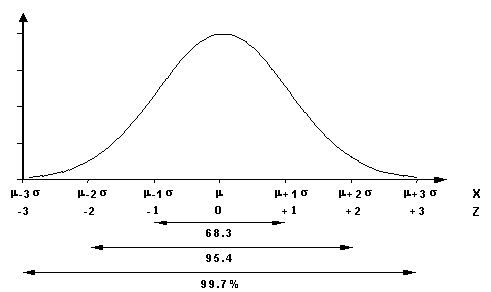The 68-95-99.7 Rules for a Normal Distribution:

*          About 68.3% of the data in a normally Distributed data set will fall within

1 standard deviation of the mean.

*          About 95.4% of the data in a normally distributed data set will fall within

2 standard deviations of the mean.

*          About 99.7% of the data in a normally distributed data set will fall within

3 standard deviations of the mean.

### Inferential data analysis

As the researcher draws scientific conclusions from his study using only a sample instead of the whole population, he can justify his conclusion with help of statistical inference tools. The principal concepts involved in statistical inference are theory of estimation and hypothesis testing.

### Theory of estimation

Point Estimation:

A single value is used to provide the best estimate of the parameter of interest.

Interval Estimation:

Interval estimates shows the estimate of the parameter and also give an idea of the confidence that the researcher has in that estimate. This leads us to consideration of confidence intervals.

# Confidence interval (CI)

A confidence interval estimate of a parameter consists of an interval, along with a probability that the interval contains the unknown parameter.  The level of confidence in a confidence interval is a probability that represents the percentage of intervals that will contain the parameter if a large number of repeated samples are obtained.  The level of confidence is denoted (1 - a)*100%.

The narrower the width of the confidence interval, the lower is the error of the point estimate it contains. The sample size, sample variance and the level of confidence all affect the width of the confidence interval.

• If the sample size increases it will decrease the width of the confidence interval.
• If the level of confidence increases the width will increase.
• If the variation in sample increase it will increases the width of confidence interval

Confidence intervals can be computed for estimating single mean and proportions and also for comparing the difference between two means or proportions. Confidence interval is widely used to represent the main clinical outcomes instead of p values as it has many advantages over it (such as giving information about effect size, variability and possible range). The most commonly used confidence interval is the 95% CI. Increasingly, medical journals and publications require authors to calculate and report the 95% CI wherever appropriate since it gives a measure of the range of effect sizes possible – information that is of great relevance to clinicians. The term 95% CI means that it is the interval within which we can be 95% sure the true population value lies. Note that the remaining 5% of the time, the value may fall outside this interval. The estimate, which is the effect size observed in the particular study is the point at which the true value is most likely to fall, though it can theoretically occur at any point within the confidence interval (or even outside it, as just alluded to).

Example:

A study is conducted to estimate the average glucose levels in patients admitted with diabetic ketoacidosis. Sample of 100 patients were selected and the mean was found to be 500 mg/dL with a 95% confidence interval of 320-780.  This means that there is a 95% chance that the true mean of all patients will lie between 320 and 780.

### Hypothesis testing vs. Estimation

Similarity:  Both use sample data to infer something about a population

Difference: Designed to answer different questions

Does a new drug lower cholesterol levels?

Measure cholesterol of 25 patients before drug & after - change in cholesterol is 15 mg/dL (225 before; 210 after)

Hypothesis test: Did the drug alter cholesterol levels?

Yes/no decision. Reject or fail to reject H0

Estimation: By how much did the drug alter cholesterol levels?

# Hypothesis testing

Setting up the Hypotheses:

The basic concept used in hypothesis testing is that it is far easier to show that something is false than to prove that it is true.

a) Two mutually exclusive & competing hypotheses:

Let us consider a situation where we want to test if a new drug is having superior efficacy to one of the standard drugs prevailing in the market for the treatment of tuberculosis. We will have to construct a null hypothesis and alternative hypothesis for this experiment as below:

1.  The “null” hypothesis (H0)

The null hypothesis indicates a neutral position (or the status quo in an interventional trial) in the given study or experiment. Typically the investigator hopes to prove this hypothesis wrong so that the alternate hypotheses (which encompasses the concept of interest to the investigator) can be accepted.

Example:

In the situation given above, though we actually want to prove the new drug to be effective, we should proceed with a neutral attitude while doing the experiment so our null hypothesis will be stated as follows:

Ho: There is no difference between the effect of new drug and standard drug in treating tuberculosis

2.  The “alternative” hypothesis (H1)

This is the hypothesis we believe or hope is true.

Example: In the above situation if we want to prove the new drug is superior then our alternative hypothesis will be:

H1:  New drug’s effect is superior to that of the standard drug.

Based on the alternative hypothesis the test will become one-tailed test or two-tailed test. Two-tailed tests are when the researcher wants to test in both the direction for the population parameter specified in the null hypothesis (i.e. either greater or lesser). If he wants to test the parameter of the null hypothesis in only one direction greater or lesser it becomes a one-tailed test.

In the above example the researcher test framed the alternative hypothesis in only one direction (new drug is superior to the standard drug) so the test becomes a one tailed test.

# b) Selecting a “significance level”: a

Significance level is the probability of rejecting the null hypothesis when it is actually true (Type I error). It is usually set at 5% i.e. a = .05 (5%)

c)      Calculate the test statistics and p value

Test statistics

Calculating the test statistics will depend on our null hypothesis. It may be testing a single mean or proportion or it may be comparing two means or proportions.

p-value

A p-value  gives the likelihood of the study effect, given that the null hypothesis is true. For example, a p-value of .03 means that, assuming that the treatment has no effect, and given the sample size, an effect as large as the observed effect would be seen in only 3% of studies.

In other words it gives the chance of observing a difference (effect) from the sample when the null hypothesis is true. For example, if get a p value of 0.02 then only a 2% chance is there for observing a difference (effect) from the sample if we assume the null hypothesis is true.

The p-value obtained in the study is evaluated against the significance level alpha. If alpha is set at .05, then a p-value of .05 or less is required to reject the null hypothesis and establish statistical significance.

d) Decision rule:

We can reject H0 if the p-value <α.

Most statistical packages calculate the p-value for a 2-tailed test. If we are conducting a 1-tailed test we must divide the p-value by 2 before deciding if it is acceptable. (In SPSS output, the p-value is labeled “Sig (2-tailed).”)

Table 1: Step by step guide to applying hypothesis testing in research

1.  Formulate a research question

2.  Formulate a research/alternative hypothesis

3.  Formulate the null hypothesis

4.  Collect data

5.  Reference a sampling distribution of the particular statistic assuming that H0

is true (in the cases so far, a sampling distribution of the mean)

6.      Decide on a significance level (a), typically .05

7.      Compute the appropriate test statistic

8.      Calculate p value

9.      Reject H0 if the p value is less than the set level of significance otherwise accept H0

Hypothesis Testing for different Situations

Testing for Single mean – Large Samples: Z-test

Z-test for single mean is useful when we want to test a sample mean against the population mean when the sample is size is large (i.e. more than 30).

Example:

A researcher wants to test the statement that the mean level of dopamine is greater than 36 in individuals with schizophrenia. He collects a sample of 54 patients with schizophrenia.

The researcher can test the hypothesis using Z-test for testing single mean.

Testing for Two means – Large Samples: Z-test for comparing two means.

Z-test for comparing two means is useful when we want to compare two sample means when the sample is size is large (i.e. more than 30).

Example:

Past studies shows that Indian men have higher cholesterol levels than Indian women. A sample of 100 males and females were taken and their cholesterol level measured – males were found to have a mean cholesterol level of 188 mg/dL and females a mean level of 164 mg/dL. Is there sufficient evidence to conclude that the males are indeed having a higher cholesterol level?

Here we can test the hypothesis using Z-test for comparing two sample means.

Testing for Single mean – t-test.

The t-test for single mean is useful when we want to test a sample mean against the population mean when the sample is size is small (i.e. less than 30).

Example:

A researcher wants to test the statement that the mean age of diabetic patients in his district is greater than 60 years. He draws a sample of 25 persons.

Here we can test the hypothesis using t-test for single mean.

Independent Sample t-test for two means.

The t-test for comparing two means is appropriate when we want to compare two independent sample means when the sample is size is small (i.e. less than 30).

Example:

A study was conducted to compare males and females in terms of average years of education with a sample of 9 females and 13 males. It was inferred that males had an average of 17 years of formal education while females had 14. Can it be concluded that males are having a higher degree of education than females within this population?

Here we can test the hypothesis using t-test for comparing two sample means.

Paired t-test for two means.

Paired t-test is useful when we want to compare the two sample means when the two sample measurements are taken from the same subject under the study like pre and post measurements.

Example:

A study was conducted to compare the effect of a drug in treating hypertension by administering it to 20 patients. BP was recorded immediately before and one hour after the drug is given.  The question of interest - is the drug effective is reducing blood pressure?

A paired t-test can be used for hypothesis testing and comparing two paired sample means.

# Testing for Single proportion: Binomial test for proportion

If we want to test a sample proportion against the population proportion we can use the

binomial test for single proportion.

Example:

A random sample of patients is recruited for a clinical study.  The researcher wants to establish that the proportion of female patients is not equal to 0.5.

The binomial test for proportion is the appropriate statistic method here.

Testing for Two proportion: Z-test for two proportions

If we want to compare two sample proportions we can use the Z-test for two proportions when the sample size is large (i.e. more than 30)

Example:

Two types of hypodermic needles, the old type and a new type, are used for giving injections. It is hoped that the new design will lead to less painful injections. The patients are allocated at random to two groups, one to receive the injections using a needle of the old type, the other to receive injections with needles of the new type.

Does the information support the belief that the proportion of patients having severe pain with injections using needles of the old type is greater than the proportion of patients with severe pain in the group getting injections using the new type?

Here we can test the hypothesis using Z-test for comparing two sample proportions.

Chi-square test (χ2)

It is a statistical procedure used to analyze categorical data.

We will explore two different types of χ2 tests:

1.  One categorical variable: Goodness-of-fit test

2.  Two categorical variables: Contingency table analysis

One categorical variable: Goodness-of-fit test

A test for comparing observed frequencies with theoretically predicted frequencies.

Two categorical variables: Contingency table analysis

Defined: a statistical procedure to determine if the distribution of one categorical variable is contingent on a second categorical variable

• Allows us to see if two categorical variables are independent from one another or are related
• Conceptually, it allows us to determine if two categorical variables are correlated

Note:

If the expected frequencies in the cells are “too small,” the χ2 test may not be valid

A conservative rule is that you should have expected frequencies of at least 5 in all cells

Example

We want to test the association between cancer and smoking habit in 250 patients. The  chi-square would be an appropriate test.

Analysis of Variance (ANOVA)

When we want to compare more than two means we will have to use an analysis of variance test.

Example:

A researcher has assembled three groups of psychology students. He teaches the same topic to each group using three different educational methodologies. The researcher wishes to determine if the three modalities are giving equivalent results. He tests all the students and records the marks obtained.

An ANOVA analysis can be used to test the hypothesis.

Multiple Comparison and Post Hoc Tests

Repeated Measures ANOVA

Repeated measures ANOVA is useful when we want to compare more than two sample means when the sample measurements are taken from the same subject enrolled in the study.

Example:

A trial was conducted to compare the effect of a drug in treating hypertension by administering it to 20 patients. BP was recorded immediately before and one, two and four hours after the drug is administered

Is the drug is effective is reducing blood pressure?

Repeated measures ANOVA would be the right way to get an answer.

Parametric Tests

The statistical hypothesis test such as z-test,t-test and ANOVA assumes the  distributions of the variables being assessed comes from a parametrized  probability distribution. The parameters usually used are the mean and standard deviation. For example, t-test assumes the variable comes from the normal population and analysis of variance assumes that the underlying distributions are normally distributed and that the variances are similar.

Parametric techniques are poweful  to detect differences or similarities than the non parametric tests

# Nonparametric/Distribution-free tests

Nonparametric tests: statistical tests that do not involve population parameters and  do not make assumptions about the shape of the population(s) from which sample(s) originate.

It is used in the following circumstances

1.  Useful when statistical assumptions have been violated

2.  Ideal for nominal (categorical) and ordinal (ranked) data

3.  Useful when sample sizes are small (as this is often when assumptions are violated)

What are the disadvantages of Nonparametric/Distribution-free tests?

1.  Tend to be less powerful than their parametric counterparts

2.  H0 & H1 not as precisely defined

There is a nonparametric/distribution-free counterpart to many parametric tests.

·         The Mann-Whitney U Test: The nonparametric counterpart of the independent samples t-test

·         The Wilcoxon Signed Rank Test: The nonparametric counterpart of the related samples t-test

·         The Kruskal-Wallis Test: The nonparametric counterpart of one-way ANOVA

Table 2: Statistical tests at a glance

 Type of variable in the study Parameters to be tested Number of variables Sample size Test Ratio variables Mean One >30 Z-test Mean Two >30 Z-test Mean One <30 t-test Mean Two <30 Independent sample t-test Mean (same subject) Two <30 Paired sample t-test Proportion One Binomial Proportion Two >30 z-test Mean More than two >30 ANOVA Mean(same subject More than two >30 Repeated measures ANOVA Nominal/ Categorical variables Association Two or more - Chi-square Ratio variables Mean Two When normality assumption violated Mann-Whitney test Ratio variables Mean (same subject) Two When normality assumption violated Wilcoxon signed rank test Ratio variables Mean Moe than Two When normality assumption violated Kruskal Wallis test

Sensitivity and Specificity

Diagnostic tests used in clinical practices have certain operating characteristics. It is important for clinicians to be aware of these test characteristics as they interpret the results of these tests, and also as they determine optimal testing strategies to get to an accurate diagnosis or assign an appropriate prognosis. Sensitivity specificity, positive predictive value and negative predictive values are key parameters used in the further evaluation of the properties of diagnostic tests. Diagnostic tests are compared to a “gold standard” that is the best single test or combination of tests that is relevant to the particular diagnosis.

Sensitivity is the chance that the diagnostic test will indicate the presence of disease when the disease is actually present.

Specificity is the chance that the diagnostic disease will indicate the absence of disease when the disease is actually absent.

Positive predictive value is the chance that a positive test result actually means that the disease is present.

Negative predictive value is the chance that a negative test result actually means that the disease is absent

Note that sensitivity depends only on the distribution of positive and negative test results within the diseased population and the specificity depends only on the distribution of the results within the non-diseased population.  They do not depend on the ratio of diseased to non-diseased and therefore are considered to be independent of disease prevalence whereas positive and negative predictive value is a function of disease prevalence and pre-test probability.

 Disease + _ Test Present True Positive (TP) False Positive (FP) Absent False Negative  (FN) True Negative (TN)

Sensitivity = TP/(TP +FN)

Specificity = TN/(TN + FP)

PPV = TP/(TP + FP)

NPV = TN/(TN + FN)

Efficiency = (TP + TN)/(TP + FP + FN + TN)

The mnemonics of “Spin” and “Snout” (adapted from those originally suggested by Sackett and colleagues) are extremely useful to remember the properties of specificity and sensitivity. A highly specific (Sp) test, if positive (p) rules “in” the disease – giving us Spin. A highly sensitive (Sn) test, if negative (n) rules “out” the disease – and there you have Snout.

# Bayes Theorem

Bayes’ theorem states the predictive value of a test will depend on the prevalence of the disease.  For diseases with high prevalence, the positive predictive value will increase and vice versa.  The negative predictive value will have an opposite effect. If a researcher uses a diagnostic test in a high prevalence setting, a positive test will be more likely to be truly positive than in a low prevalence setting.

# ROC curves

ROC curves illustrate the trade-off in sensitivity for specificity. The greater the area under the ROC curve, the better the overall trade-off between sensitivity and specificity. This is a more sophisticated way to determine the optimal points for weighing sensitivity versus specificity since we know that if one is increased, the other invariably tends to decrease.

Relative Risk (RR):

Probability of the disease if the risk factor is present divided by the probability of the disease if the risk factor is absent.  Example:  a study to evaluate the relationship between a food habit and diabetic might compare a group of People with the specific food habit to a group not on the food habit and follow them for the development of diabetic.  If 10% of the people on the food habit developed diabetic and 0.5% of the people not on the food habit developed it, the relative risk would be 20.

Relative risk of 1: no effect

Relative risk >1: positive effect

Relative risk <1: negative effect

Relative risk should be presented with confidence intervals (CI), which to reflect a statistically significant finding, should not contain data points that include an RR of 1. Conversely, it can be seen that if the RR CI does include 1, then the RR is not statistically significant.

In the food habit /diabetic example If p value was 0.05 and the 95% confidence interval for the relative risk of 20 was 0.7-25, then statistical significance would not be achieved since the range of values includes 1.

Odds Ratio (OR):  similar to relative risk, but used for case-control studies.  The odds of having the risk factor if the disease is present divided by the odds of having the risk factor if the disease is absent gives us the OR.

Likelihood Ratio (LR)

Likelihood ratios are very useful in that they are an indication of the degree to which a test result will change the pre-test probability of disease.

It can be calculated in two ways one is for a positive result and another is for a negative result.

For a given test, to get a positive likelihood ratio, the probability of a positive test result if the disease is present divided by the probability of a positive test result if the disease is absent.

+LR = sensitivity/(1-specificity)

Probability of a negative test result if the disease is present divided by the probability of a negative test result if the disease is absent to get a negative likelihood ratio.

-LR = (1-sensitivity)/specificity

LR=1: no effect on pre-test probability

LR>1:  positive effect

LR<1:  negative effect

LR=1-2 or 0.5-1:  minimal effect

LR=2-5 or 0.2-0.5:  small effect

LR=5-10 or 0.1-0.2:  moderate effect

LR>10 or <0.1:  large effect

Statistical Software

The life of medical researchers (and biostatisticans) have been greatly simplified by the ready availability of excellent free as well as commercial software. Commercial software such SAS, SPSS and STATA are widely used while free software such as Epi-info are also extremely useful in statistical analysis. Link for the free statistical packages is given in the recommended resources

# Survival analysis

Survival analysis is a form of time to event analysis. In other words it is defined as measuring the time between an origin point and an end point, often the end point will be taken as death of the patient, occurrence of symptoms or disease onset in clinical research.

Aims of survival analysis may be to estimate survival, compare survival times between two groups or know the relationship of the explanatory variables to the survival time.

Survival analysis involves concepts of censoring in estimating the survival times.

Censoring:

Censoring is defined as study of incomplete observations of the survival time. The following are the types of censoring used in the survival analysis.

Right censoring:

Some individuals may not be observed for the full time to failure, e.g. because of loss to follow-up, drop out from the study and termination of the study

Interval Censoring:

This occurs when we do not know the exact time of failure, but rather have data on two time points between which the event occurred.

Left censoring:

This occurs when some subjects have a delayed entry into the study.

# Kaplan Meier Curve

This curve is used to estimate the survival time and also interpret and compare the two groups survival times. An example is show below: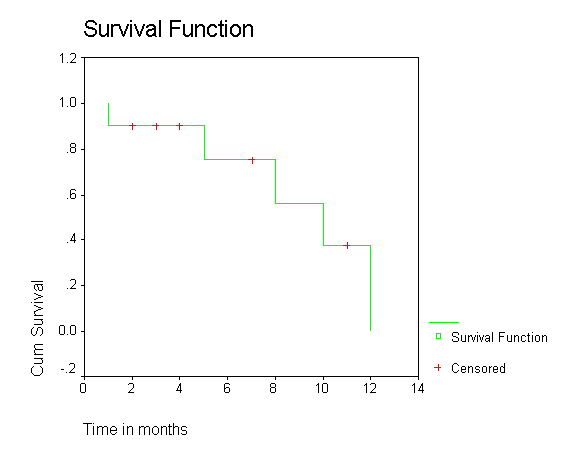Cox regression model:

It is used to assess the relation between the explanatory variables and survival times.

Multivariate Analysis

When we want to analyse more than two variables simultaneously we can use multivariate analysis.

Multivariate Analysis Techniques are

• Multiple Regression
• Multivariate analysis of variance (MANOVA)
• Multivariate analysis of covariance (MANCOVA)
• Canonical correlation analysis
• Discriminant function analysis
• Cluster analysis
• Factor analysis
• Correspondence analysis

Discussing them in detail will be beyond the scope of this chapter. The interested reader can consult the recommended resources for further insight.

Meta-analysis

Meta analysis will be used to combine results of similar studies quantitatively to give a over all picture of the problem undertaken. One has to have explicit criteria for inclusion to avoid combining studies that have fundamental differences in design. The strengths of a meta-analysis is that it functions essentially like a mega-study with increase in sample size and greater generalizability since data is being captured from studies conducted at multiple sites, by differing groups of investigators.

To start a meta analysis we will have identify the articles available with respect to the problem we have undertaken and then combine the articles which meet our pre-determined inclusion criteria.

Recommended resources

Chatfield C, Collin A. J. Introduction to multivariate analysis. First edition, Chapman and Hall, London; 1980

Flury, B, Riedwyl, H. Multivariate statistics: A practical approach. Chapman and Hall 1988

Hyperstat Online at

Sackett DL, Haynes RB, Guyatt GH, Tugwell P. Clinical Epidemiology: A Basic
Science for Clinical Medicine. 2nd ed. Boston, Mass: Little Brown & Co Inc;
1991:145-148.